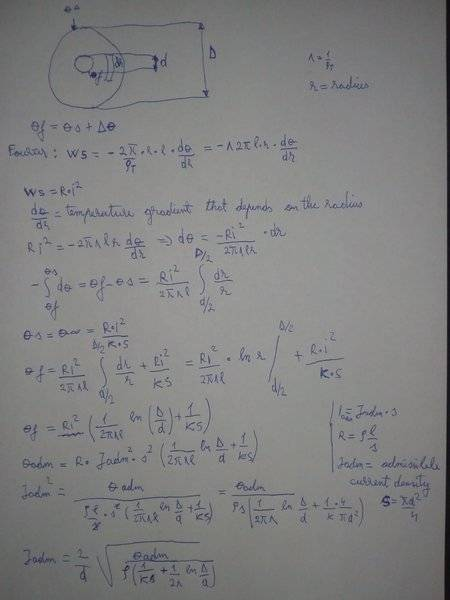# Heat loss in a conductor based on Fourier's law

## Homework Statement

Find the admissible current density Jadm for a wire that has no insulation and also for a wire that has two layers of insulation and compare it to Jadm for the case when the wire has only one layer of insulation.

2. The attempt at a solution and equations
In the image I've managed to find Jadm for the case with one layer of insulation, but for the other cases I don't know how to exactly modify the terms in order to obtain the jadm values. Corrections: s=(π*d^2)/4 , S=π*D*l,#### Attachments

Last edited:

haruspex
Homework Helper
Gold Member
For the uninsulated case you will need to consider the temperature gradient within the wire. This is more complicated because the heat generation is distributed over the cross section. Strictly speaking, the resistance will depend on the temperature, but that makes it really complicated.
And to be exact, you would need to consider the gradient in the wire in the insulated cases too, but it probably becomes insignificant.

For two layers of insulation, I don't see the difficulty. Why isn't that just doubling the thickness?

•Cassius1n
I think the temperature gradient in the cross section of the conductor itself may be neglected so θf is the entire conductor temperature.
Since θs=R*I^2/(K*S)+θa where θa=ambient [air] temperature
then θf=R*I^2*(1/(2*π*λ*l*ln(D/d)+1/(K*S)+θa)
In case of uninsulated conductor R*I^2=K*S*(θf-θa)
R depends on θf [ R=ρo*[1+α*( θf-20)]*lngth/scu
ρo= conductor resistivity at 20oC
α=0.00403 for aluminum and 0.00393 for copper.
K depends on exterior surface type [considering heat evacuation by convection and radiation together].

•Cassius1n
haruspex
Homework Helper
Gold Member
In case of uninsulated conductor R*I^2=K*S*(θf-θa)
What are K and S here?

If someone intends to actually calculate the admissible current density -or at least the allowable current [ampacity or current carrying capacity] he has to follow Nehrer and McGrath method or IEC 60287-1-1 and 2-1.
From IEC 60287-2-1 for a cable in open air K[=T4*π*d]=1/h/∆s^(1/4) where h=Z/d^g+E
From Table 2 for a single cable Z=0.21 E=3.94 g=0.6 h=0.21/d^0.6+3.94
∆s=is the excess of cable surface temperature above ambient temperature
S=π*d*length

•Cassius1n
For bare conductor the steady state current could be calculated according to IEEE 738/2006 formula 1(b) :
I=SQRT((qc+qr-qs)/R(T)) where :
qc=Convected heat loss rate per unit length[W/m]
qr=Radiated heat loss rate per unit length[W/m]
qs=Heat gain rate from sun[W/m]
For natural convection [no wind]:
qc = 0.0205*ρf^0.5*D^0.75*(Tc −Ta)^1.25
qr=0.0178*D*ε*{[(Tc+273)/100]^4-[(Ta+273)/100]^4}
qs =α*Qse*sin(θ)*A'
where:
D=Conductor diameter[mm]
pf=Density of air[kg/m^3]
Tc=Conductor temperature[oC]
Ta=Ambient air temperature[oC]
ε=Emissivity (0.23 to 0.91)
α=Solar absorptivity (0.23 to 0.91)
A'=Projected area of conductor per unit length[m^2/m]
θ=Effective angle of incidence of the sun’s rays[degrees]
Qse=Total solar and sky radiated heat flux rate elevation corrected [W/m^2]
Let's say the conductor is a solid copper of 50 mm^2 cross section area [D= 7.8 mm ε=0.5 Tc=80oC no wind, no sun,Ta=40oC]
qc=10.19 W; qr=4,12 W; I=172.9 A; R(80Oc)=0.000478 ohm/m R*I^2=14.3 W/m; S=0.0245 m^2
Then K= 14.3/0.0245=584 W/m^2

•Cassius1n
Thank you for the help!